•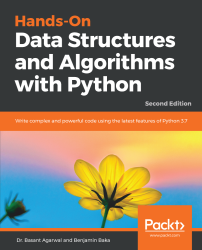#### Hands-On Data Structures and Algorithms with Python - Second Edition#### Overview of this book

Data structures allow you to store and organize data efficiently. They are critical to any problem, provide a complete solution, and act like reusable code. Hands-On Data Structures and Algorithms with Python teaches you the essential Python data structures and the most common algorithms for building easy and maintainable applications. This book helps you to understand the power of linked lists, double linked lists, and circular linked lists. You will learn to create complex data structures, such as graphs, stacks, and queues. As you make your way through the chapters, you will explore the application of binary searches and binary search trees, along with learning common techniques and structures used in tasks such as preprocessing, modeling, and transforming data. In the concluding chapters, you will get to grips with organizing your code in a manageable, consistent, and extendable way. You will also study how to bubble sort, selection sort, insertion sort, and merge sort algorithms in detail. By the end of the book, you will have learned how to build components that are easy to understand, debug, and use in different applications. You will get insights into Python implementation of all the important and relevant algorithms.
PrefaceFree Chapter
Python Objects, Types, and ExpressionsPython Data Types and StructuresPrinciples of Algorithm DesignLists and Pointer StructuresStacks and QueuesTreesHashing and Symbol TablesGraphs and Other AlgorithmsSearchingSortingSelection AlgorithmsString Algorithms and TechniquesDesign Techniques and StrategiesImplementations, Applications, and ToolsOther Books You May Enjoy# Overview of data types and objects

Python contains various built-in data types. These include four numeric types (int, float, complex, bool), four sequence types (str, list, tuple, range), one mapping type (dict), and two set types. It is also possible to create user-defined objects, such as functions or classes. We will look at the string and the list data types in this chapter and the remaining built-in types in the next chapter.

All data types in Python are objects. In fact, pretty much everything is an object in Python, including modules, classes, and functions, as well as literals such as strings and integers. Each object in Python has a type, a value, and an identity. When we write greet= "helloworld", we are creating an instance of a string object with the value "hello world" and the identity of greet. The identity of an object acts as a pointer to the object's location in memory. The type of an object, also known as the object's class, describes the object's internal representation, as well as the methods and operations it supports. Once an instance of an object is created, its identity and type cannot be changed.

We can get the identity of an object by using the built-in function id(). This returns an identifying integer and on most systems, this refers to its memory location, although you should not rely on this in any of your code.

Also, there are a number of ways to compare objects; for example, see the following:

`if a==b:    # a and b have the same valueif a is b:    # if a and b are the same objectif type(a) is type(b):   #a and b are the same type`

An important distinction needs to be made between mutable and immutable objects. Mutable objects such as lists can have their values changed. They have methods, such as insert() or append(), that change an object's value. Immutable objects such as strings cannot have their values changed, so when we run their methods, they simply return a value rather than change the value of an underlying object. We can, of course, use this value by assigning it to a variable or using it as an argument in a function. For example, the int class is immutable—once an instance of it is created, its value cannot be changed, however, an identifier referencing this object can be reassigned another value.

# Strings

Strings are immutable sequence objects, with each character representing an element in the sequence. As with all objects, we use methods to perform operations. Strings, being immutable, do not change the instance; each method simply returns a value. This value can be stored as another variable or given as an argument to a function or method.

The following table is a list of some of the most commonly used string methods and their descriptions:

 Method Description s.capitalize Returns a string with only the first character capitalized, the rest remaining lowercase. s.count(substring,[start,end]) Counts occurrences of a substring. s.expandtabs([tabsize]) Replaces tabs with spaces. s.endswith(substring,[start, end] Returns True if a string ends with a specified substring. s.find(substring,[start,end]) Returns index of first presence of a substring. s.isalnum() Returns True if all chars are alphanumeric of string s. s.isalpha() Returns True if all chars are alphabetic of string s. s.isdigit() Returns True if all chars are digits in the string. s.split([separator],[maxsplit]) Splits a string separated by whitespace or an optional separator. Returns a list. s.join(t) Joins the strings in sequence t. s.lower() Converts the string to all lowercase. s.replace(old, new[maxreplace]) Replaces old substring with a new substring. s.startswith(substring, [start, end]]) Returns True if the string starts with a specified substring. s.swapcase() Returns a copy of the string with swapped case in the string. s.strip([characters]) Removes whitespace or optional characters. s.lstrip([characters]) Returns a copy of the string with leading characters removed.

Strings, like all sequence types, support indexing and slicing. We can retrieve any character from a string by using its index s[i]. We can retrieve a slice of a string by using s[i:j], where i and j are the start and end points of the slice. We can return an extended slice by using a stride, as in the following—s[i:j:stride]. The following code should make this clear: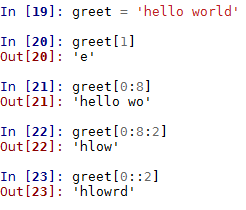The first two examples are pretty straightforward, returning the character located at index 1 and the first seven characters of the string, respectively. Notice that indexing begins at 0. In the third example, we are using a stride of 2. This results in every second character being returned. In the final example, we omit the end index and the slice returns every second character in the entire string.

You can use any expression, variable, or operator as an index as long as the value is an integer: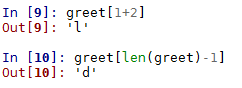Another common operation is traversing through a string with a loop: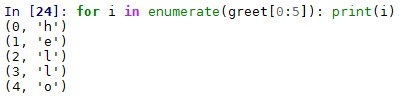Given that strings are immutable, a common question that arises is how we perform operations such as inserting values. Rather than changing a string, we need to think of ways to build new string objects for the results we need. For example, if we wanted to insert a word into our greeting, we could assign a variable to the following: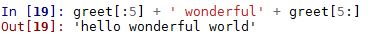As this code shows, we use the slice operator to split the string at index position 5 and use + to concatenate. Python never interprets the contents of a string as a number. If we need to perform mathematical operations on a string, we need to first convert them to a numeric type: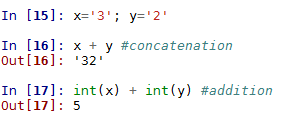# Lists

List is one of the most commonly used built-in data structures, as they can store any number of different data types. They are simple representations of objects and are indexed by integers starting from zero, as we saw in the case of strings.

The following table contains the most commonly used list methods and their descriptions:

 Method Description list(s) Returns a list of sequence s. s.append(x) Appends element x at the end of list s. s.extend(x) Appends list x at the end of list s. s.count(x) Returns the count of the occurrence of x in list s. s.index(x,[start],[stop]) Returns the smallest index i, where s[i]==x. We can include an optional start and stop index for the lookup. s.insert(i,e) Inserts x at index i. s.pop(i) Returns the element i and removes it from the list s. s.remove(x) Removes element x from the list s. s.reverse() Reverses the order of list s. s.sort(key,[reverse]) Sorts list s with optional key and reverses it.

In Python, lists implementation is different when compared to other languages. Python does not create multiple copies of a variable. For example, when we assign a value of one variable in another variable, both variables point to the same memory address where the value is stored. A copy would only be allocated if the variables change their values. This feature makes Python memory efficient, in the sense that it only creates multiple copies when it is required.

This has important consequences for mutable compound objects such as lists. Consider the following code: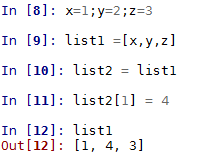In the preceding code, both the list1 and list2 variables are pointing to the same memory location. However, when we change the y through list2 to 4, we are actually changing the same y variable that list1 is pointing to as well.

An important feature of list is that it can contain nested structures; that is, list can contain other lists. For example, in the following code, list items contains three other lists: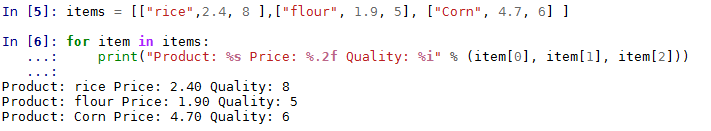We can access the values of the list using the bracket operators and, since lists are mutable, they are copied in place. The following example demonstrates how we can use this to update elements; for example, here we are raising the price of flour by 20 percent:We can create a list from expressions using a very common and intuitive method; that is, list comprehensions. It allows us to create a list through an expression directly into the list. Consider the following example, where a list l is created using this expression: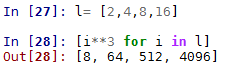List comprehensions can be quite flexible; for example, consider the following code. It essentially shows two different ways to performs a function composition, where we apply one function (x*4) to another (x*2). The following code prints out two lists representing the function composition of f1 and f2, calculated first using a for loop and then using a list comprehension:

`def f1(x): return x*2 def f2(x): return x*4lst=[]for i in range(16):   lst.append(f1(f2(i)))print(lst)print([f1(x) for x in range(64) if x in [f2(j) for j in range(16)]])`

The first line of output is from the for loop construct. The second is from the list comprehension expression: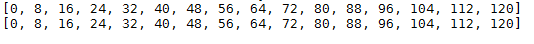List comprehensions can also be used to replicate the action of nested loops in a more compact form. For example, we multiply each of the elements contained within list1 with each other: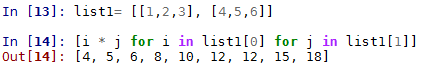We can also use list comprehensions with other objects such as strings, to build more complex structures. For example, the following code creates a list of words and their letter count: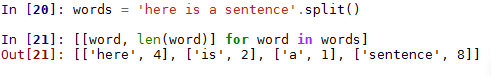As we will see, lists form the foundation of many of the data structures we will look at. Their versatility, ease of creation, and use enable them to build more specialized and complex data structures.

# Functions as first class objects

In Python, it is not only data types that are treated as objects. Both functions and classes are what are known as first class objects, allowing them to be manipulated in the same ways as built-in data types. By definition, first class objects are the following:

• Created at runtime
• Assigned as a variable or in a data structure
• Passed as an argument to a function
• Returned as the result of a function

In Python, the term first class object is a bit of a misnomer, since it implies some sort of hierarchy, whereas all Python objects are essentially first class.

To have a look at how this works, let's define a simple function:

`def greeting(language):    if language=='eng':         return 'hello world'      if language =='fr'        return 'Bonjour le monde'      else: return  'language not supported'`

Since user-defined functions are objects, we can do things such as include them in other objects, such as lists: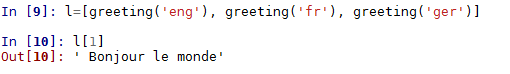Functions can also be used as arguments for other functions. For example, we can define the following function: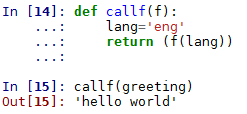Here, callf() takes a function as an argument, sets a language variable to 'eng', and then calls the function with the language variable as its argument. We could see how this would be useful if, for example, we wanted to produce a program that returns specific sentences in a variety of languages, perhaps for some sort of natural language application. Here, we have a central place to set the language. As well as our greeting function, we could create similar functions that return different sentences. By having one point where we set the language, the rest of the program logic does not have to worry about this. If we want to change the language, we simply change the language variable and we can keep everything else the same.

# Higher order functions

Functions that take other functions as arguments, or that return functions, are called higher order functions. Python 3 contains two built-in higher order functions—filter() and map(). Note that in earlier versions of Python, these functions returned lists; in Python 3, they return an iterator, making them much more efficient. The map() function provides an easy way to transform each item into an iterable object. For example, here is an efficient, compact way to perform an operation on a sequence. Note the use of the lambda anonymous function: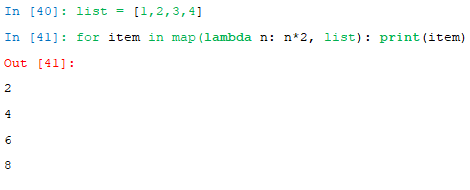Similarly, we can use the filter built-in function to filter items in a list: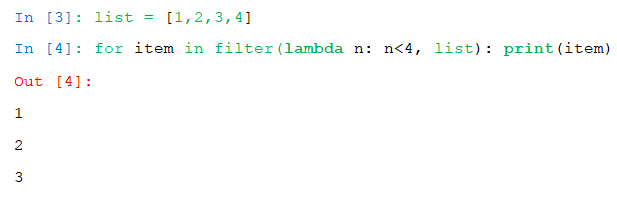Note that both map and filter perform the same function similar to what can be achieved by list comprehensions. There does not seem to be a great deal of difference in the performance characteristics, apart from a slight performance advantage when using the in-built functions map and filter without the lambda operator, compared to list comprehensions. Despite this, most style guides recommend the use of list comprehensions over built-in functions, possibly because they tend to be easier to read.

Creating our own higher order functions is one of the hallmarks of functional programming style. A practical example of how higher order functions can be useful is demonstrated by the following. Here, we are passing the len function as the key to the sort function. This way, we can sort a list of words by length: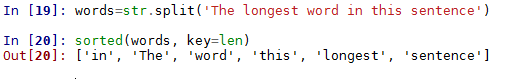Here is another example for case-insensitive sorting: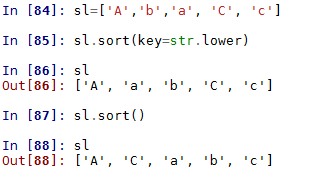Note the difference between the list.sort() method and the sorted built-in function. The list.sort() method, a method of the list object, sorts the existing instance of a list without copying it. This method changes the target object and returns None. It is an important convention in Python that functions or methods that change the object return None, to make it clear that no new object was created and that the object itself was changed.

On the other hand, the sorted built-in function returns a new list. It actually accepts any iterable object as an argument, but it will always return a list. Both list sort and sorted take two optional keyword arguments as key.

A simple way to sort more complex structures is to use the index of the element to sort, using the lambda operator, for example: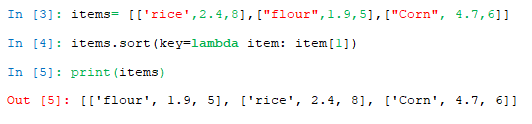Here we have sorted the items by price.

# Recursive functions

Recursion is one of the most fundamental concepts of computer science. It is called recursion when a function takes one or more calls to itself during execution. Loop iterations and recursion are different in the sense that loops execute statements repeatedly through a Boolean condition or through a series of elements, whereas recursion repeatedly calls a function. In Python, we can implement a recursive function simply by calling it within its own function body. To stop a recursive function turning into an infinite loop, we need at least one argument that tests for a terminating case to end the recursion. This is sometimes called the base case. It should be pointed out that recursion is different from iteration. Although both involve repetition, iteration loops through a sequence of operations, whereas recursion repeatedly calls a function. Technically, recursion is a special case of iteration known as tail iteration, and it is usually always possible to convert an iterative function to a recursive function and vice versa. The interesting thing about recursive functions is that they are able to describe an infinite object within a finite statement.

The following code should demonstrate the difference between recursion and iteration. Both these functions simply print out numbers between low and high, the first one using iteration and the second using recursion: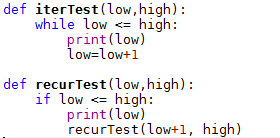Notice that for iterTest, the iteration example, we use a while statement to test for the condition, then call the print method, and finally increment the low value. The recursive example tests for the condition, prints, then calls itself, incrementing the low variable in its argument. In general, iteration is more efficient; however, recursive functions are often easier to understand and write. Recursive functions are also useful for manipulating recursive data structures such as linked lists and trees, as we will see.